# PHYSICAL WORLD part 2

3. Sep 2021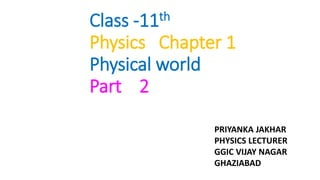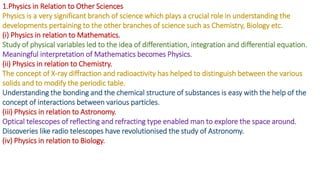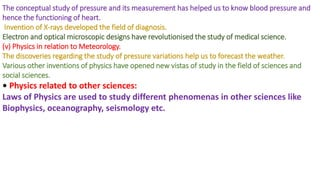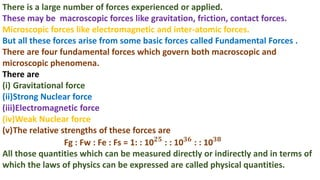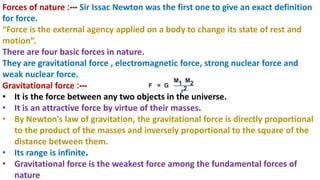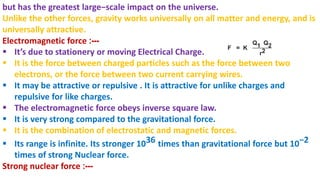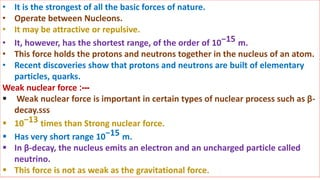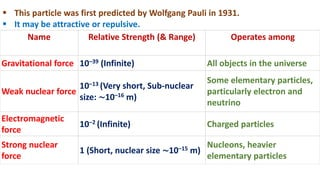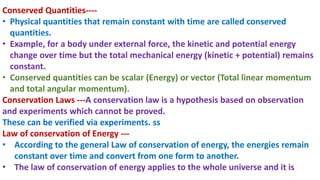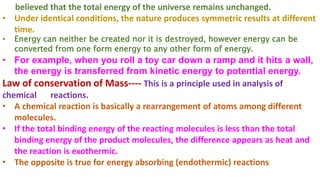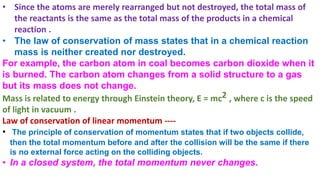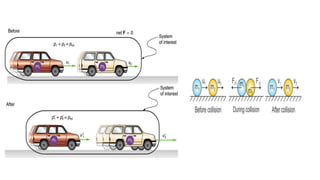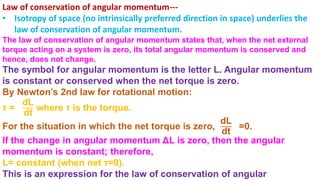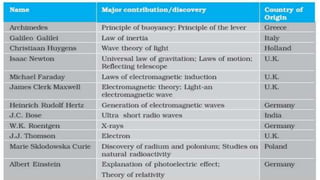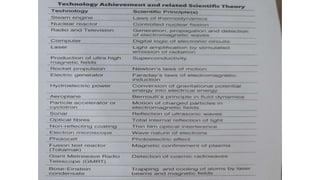1 von 15

### PHYSICAL WORLD part 2

• 1. Class -11th Physics Chapter 1 Physical world Part 2 PRIYANKA JAKHAR PHYSICS LECTURER GGIC VIJAY NAGAR GHAZIABAD
• 2. 1.Physics in Relation to Other Sciences Physics is a very significant branch of science which plays a crucial role in understanding the developments pertaining to the other branches of science such as Chemistry, Biology etc. (i) Physics in relation to Mathematics. Study of physical variables led to the idea of differentiation, integration and differential equation. Meaningful interpretation of Mathematics becomes Physics. (ii) Physics in relation to Chemistry. The concept of X-ray diffraction and radioactivity has helped to distinguish between the various solids and to modify the periodic table. Understanding the bonding and the chemical structure of substances is easy with the help of the concept of interactions between various particles. (iii) Physics in relation to Astronomy. Optical telescopes of reflecting and refracting type enabled man to explore the space around. Discoveries like radio telescopes have revolutionised the study of Astronomy. (iv) Physics in relation to Biology.
• 3. The conceptual study of pressure and its measurement has helped us to know blood pressure and hence the functioning of heart. Invention of X-rays developed the field of diagnosis. Electron and optical microscopic designs have revolutionised the study of medical science. (v) Physics in relation to Meteorology. The discoveries regarding the study of pressure variations help us to forecast the weather. Various other inventions of physics have opened new vistas of study in the field of sciences and social sciences. • Physics related to other sciences: Laws of Physics are used to study different phenomenas in other sciences like Biophysics, oceanography, seismology etc.
• 4. There is a large number of forces experienced or applied. These may be macroscopic forces like gravitation, friction, contact forces. Microscopic forces like electromagnetic and inter-atomic forces. But all these forces arise from some basic forces called Fundamental Forces . There are four fundamental forces which govern both macroscopic and microscopic phenomena. There are (i) Gravitational force (ii)Strong Nuclear force (iii)Electromagnetic force (iv)Weak Nuclear force (v)The relative strengths of these forces are Fg : Fw : Fe : Fs = 1: : 10𝟐𝟓 : : 10𝟑𝟔 : : 10𝟑𝟖 All those quantities which can be measured directly or indirectly and in terms of which the laws of physics can be expressed are called physical quantities.
• 5. Forces of nature :--- Sir Issac Newton was the first one to give an exact definition for force. “Force is the external agency applied on a body to change its state of rest and motion”. There are four basic forces in nature. They are gravitational force , electromagnetic force, strong nuclear force and weak nuclear force. Gravitational force :--- • It is the force between any two objects in the universe. • It is an attractive force by virtue of their masses. • By Newton’s law of gravitation, the gravitational force is directly proportional to the product of the masses and inversely proportional to the square of the distance between them. • Its range is infinite. • Gravitational force is the weakest force among the fundamental forces of nature F = G M1 M2 r2
• 6. but has the greatest large−scale impact on the universe. Unlike the other forces, gravity works universally on all matter and energy, and is universally attractive. Electromagnetic force :---  It’s due to stationery or moving Electrical Charge.  It is the force between charged particles such as the force between two electrons, or the force between two current carrying wires.  It may be attractive or repulsive . It is attractive for unlike charges and repulsive for like charges.  The electromagnetic force obeys inverse square law.  It is very strong compared to the gravitational force.  It is the combination of electrostatic and magnetic forces.  Its range is infinite. Its stronger 1036 times than gravitational force but 10−2 times of strong Nuclear force. Strong nuclear force :--- F = K Q1 Q2 r2
• 7. • It is the strongest of all the basic forces of nature. • Operate between Nucleons. • It may be attractive or repulsive. • It, however, has the shortest range, of the order of 10−15 m. • This force holds the protons and neutrons together in the nucleus of an atom. • Recent discoveries show that protons and neutrons are built of elementary particles, quarks. Weak nuclear force :---  Weak nuclear force is important in certain types of nuclear process such as β- decay.sss  10−13 times than Strong nuclear force.  Has very short range 10−15 m.  In β-decay, the nucleus emits an electron and an uncharged particle called neutrino.  This force is not as weak as the gravitational force.
• 8. Name Relative Strength (& Range) Operates among Gravitational force 10–39 (Infinite) All objects in the universe Weak nuclear force 10–13 (Very short, Sub-nuclear size: ∼10–16 m) Some elementary particles, particularly electron and neutrino Electromagnetic force 10‒2 (Infinite) Charged particles Strong nuclear force 1 (Short, nuclear size ∼10–15 m) Nucleons, heavier elementary particles  This particle was first predicted by Wolfgang Pauli in 1931.  It may be attractive or repulsive.
• 9. Conserved Quantities---- • Physical quantities that remain constant with time are called conserved quantities. • Example, for a body under external force, the kinetic and potential energy change over time but the total mechanical energy (kinetic + potential) remains constant. • Conserved quantities can be scalar (Energy) or vector (Total linear momentum and total angular momentum). Conservation Laws ---A conservation law is a hypothesis based on observation and experiments which cannot be proved. These can be verified via experiments. ss Law of conservation of Energy --- • According to the general Law of conservation of energy, the energies remain constant over time and convert from one form to another. • The law of conservation of energy applies to the whole universe and it is
• 10. believed that the total energy of the universe remains unchanged. • Under identical conditions, the nature produces symmetric results at different time. • Energy can neither be created nor it is destroyed, however energy can be converted from one form energy to any other form of energy. • For example, when you roll a toy car down a ramp and it hits a wall, the energy is transferred from kinetic energy to potential energy. Law of conservation of Mass---- This is a principle used in analysis of chemical reactions. • A chemical reaction is basically a rearrangement of atoms among different molecules. • If the total binding energy of the reacting molecules is less than the total binding energy of the product molecules, the difference appears as heat and the reaction is exothermic. • The opposite is true for energy absorbing (endothermic) reactions
• 11. • Since the atoms are merely rearranged but not destroyed, the total mass of the reactants is the same as the total mass of the products in a chemical reaction . • The law of conservation of mass states that in a chemical reaction mass is neither created nor destroyed. For example, the carbon atom in coal becomes carbon dioxide when it is burned. The carbon atom changes from a solid structure to a gas but its mass does not change. Mass is related to energy through Einstein theory, E = mc2 , where c is the speed of light in vacuum . Law of conservation of linear momentum ---- • The principle of conservation of momentum states that if two objects collide, then the total momentum before and after the collision will be the same if there is no external force acting on the colliding objects. • In a closed system, the total momentum never changes.
• 13. Law of conservation of angular momentum--- • Isotropy of space (no intrinsically preferred direction in space) underlies the law of conservation of angular momentum. The law of conservation of angular momentum states that, when the net external torque acting on a system is zero, its total angular momentum is conserved and hence, does not change. The symbol for angular momentum is the letter L. Angular momentum is constant or conserved when the net torque is zero. By Newton’s 2nd law for rotational motion: τ = dL dt where τ is the torque. For the situation in which the net torque is zero, dL dt =0. If the change in angular momentum ΔL is zero, then the angular momentum is constant; therefore, L= constant (when net τ=0). This is an expression for the law of conservation of angular